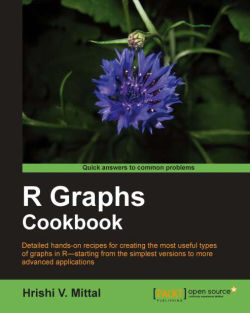### R Graphs Cookbook

By Hrishi V. Mittal
• Start FREE trial Subscribe Access now
• \$29.99 eBook

• Constantly updated with 100+ new titles each month
• Breadth and depth in over 1,000+ technologies

With more than two million users worldwide, R is one of the most popular open source projects. It is a free and robust statistical programming environment with very powerful graphical capabilities. Analyzing and visualizing data with R is a necessary skill for anyone doing any kind of statistical analysis, and this book will help you do just that in the easiest and most efficient way possible.

Unlike other books on R, this book takes a practical, hands-on approach and you dive straight into creating graphs in R right from the very first page.

You want to harness the power of this open source programming language to visually present and analyze your data in the best way possible – and this book will show you how.

The R Graph Cookbook takes a practical approach to teaching how to create effective and useful graphs using R. This practical guide begins by teaching you how to make basic graphs in R and progresses through subsequent dedicated chapters about each graph type in depth. It will demystify a lot of difficult and confusing R functions and parameters and enable you to construct and modify data graphics to suit your analysis, presentation, and publication needs.

You will learn all about making graphics such as scatter plots, line graphs, bar charts, pie charts, dot plots, heat maps, histograms and box plots. In addition, there are detailed recipes on making various combinations and advanced versions of these graphs. Dedicated chapters on polishing and finalizing graphs will enable you to produce professional-quality graphs for presentation and publication. With R Graph Cookbook in hand, making graphs in R has never been easier.

Publication date:
January 2011
Publisher
Packt
Pages
272
ISBN
9781849513067

## Chapter 1. Basic Graph Functions

In this chapter, we will cover the following recipes:

• Creating scatter plots

• Creating line graphs

• Creating bar charts

• Creating histograms and density plots

• Creating box plots

• Adjusting X and Y axis limits

• Creating heat maps

• Creating pairs plots

• Creating multiple plot matrix layouts

• Creating graphs with maps

• Saving and exporting graphs

## Introduction

In this chapter, we will see how to use R to make some very basic types of graphs, which are likely to be used in almost any kind of analysis. The recipes in this chapter will give you a feel for how much can be accomplished with very little R code, which is one big reason why R is a good choice for an analysis platform.

Although the examples in this chapter are of a basic nature, we will go through all the steps to get you going from reading your data into R, making a first graph, tweaking it to suit your needs, and then saving and exporting it for use in presentations and publications.

First and foremost, you need to download and install R on your computer. All R packages are hosted on the﻿ Comprehensive R Archive Network or CRAN (http://cran.r-project.org/). R is available for all the three major operating systems at the following locations on the web:

### Note

Please read the FAQs (http://cran.r-project.org/faqs.html) and manuals (http://cran.r-project.org/manuals.html) on the CRAN site for detailed help on installation.

Just having the base installation of R should set you up for all the recipes in this book.

Please note that the R code in this book has some comments explaining the code. Any text on a line following the` #` symbol is treated by R as a comment. For example, you may see something like this:

```col="yellow" #Setting the color to yellow
```

As you can see clearly, the text after the` #` explains what the code is doing. Setting the color to yellow in this case. Comments are a way of documenting code so that others reading your code can understand it better. It also serves to help you and you can also understand your code better when you come back to it after a long period of time. Please read each line of code carefully and look out for any comments that will help you understand the code better.

## Introduction

In this chapter, we will see how to use R to make some very basic types of graphs, which are likely to be used in almost any kind of analysis. The recipes in this chapter will give you a feel for how much can be accomplished with very little R code, which is one big reason why R is a good choice for an analysis platform.

Although the examples in this chapter are of a basic nature, we will go through all the steps to get you going from reading your data into R, making a first graph, tweaking it to suit your needs, and then saving and exporting it for use in presentations and publications.

First and foremost, you need to download and install R on your computer. All R packages are hosted on the﻿ Comprehensive R Archive Network or CRAN (http://cran.r-project.org/). R is available for all the three major operating systems at the following locations on the web:

### Note

Please read the FAQs (http://cran.r-project.org/faqs.html) and manuals (http://cran.r-project.org/manuals.html) on the CRAN site for detailed help on installation.

Just having the base installation of R should set you up for all the recipes in this book.

Please note that the R code in this book has some comments explaining the code. Any text on a line following the` #` symbol is treated by R as a comment. For example, you may see something like this:

```col="yellow" #Setting the color to yellow
```

As you can see clearly, the text after the` #` explains what the code is doing. Setting the color to yellow in this case. Comments are a way of documenting code so that others reading your code can understand it better. It also serves to help you and you can also understand your code better when you come back to it after a long period of time. Please read each line of code carefully and look out for any comments that will help you understand the code better.

## Creating scatter plots

This recipe describes how to make scatter plots using some very simple commands. We'll go from a single line of code, which makes a scatter plot from pre-loaded data, to a script of a few lines that produces a scatter plot customized with colors, titles, and axes limits specified by us.

All you need to do to get started is start R. You should have the R prompt on your screen as shown in the following screenshot: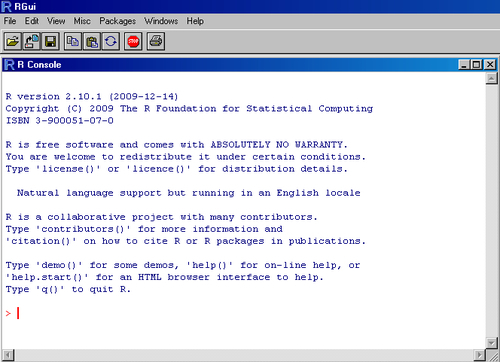### How to do it...

Let's use one of R's inbuilt datasets called` cars` to look at the relationship between the speed of cars and the distances taken to stop (recorded in the 1920s).

To make your first scatter plot, type the following command at the R prompt:

```plot(cars\$dist~cars\$speed)
```

This should bring up a window with the following graph showing the relationship between the distance travelled by cars plotted with their speeds: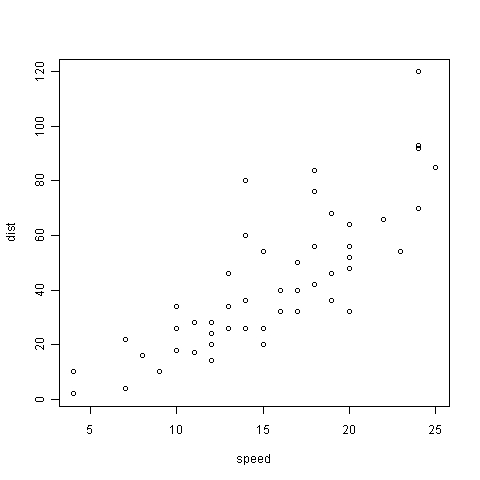Now, let's tweak the graph to make it look better. Type the following code at the R prompt:

```plot(cars\$dist~cars\$speed, # y~x
main="Relationship between car distance & speed", # Plot Title
xlab="Speed (miles per hour)", #X axis title
ylab="Distance travelled (miles)", #Y axis title
xlim=c(0,30), #Set x axis limits from 0 to 30
ylim=c(0,140), #Set y axis limits from 0 to 140
xaxs="i", #Set x axis style as internal
yaxs="i", #Set y axis style as internal
col="red", #Set the color of plotting symbol to red
pch=19) #Set the plotting symbol to filled dots
```

This should produce the following result: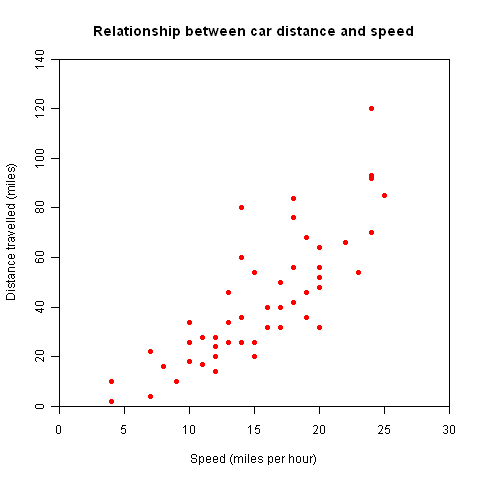### How it works...

R comes preloaded with many datasets. In the example, we used one such dataset called` cars`, which has two columns of data, with the names` speed` and` dist`. To see the data, simply type cars at the R prompt and press Enter:

```>cars
speed dist
1 4 2
2 4 10
3 7 4
4 7 22
. . .
47 24 92
48 24 93
49 24 120
50 25 85
>
```

As the output from the R command line shows, the` cars` dataset has two columns and 50 rows of data.

The` plot()` command is the simplest way to make scatter plots (and other types of plots as we'll see in a moment).

In the first example, we simply pass the` x` and` y` arguments that we want to plot in the form` plot(y~x)` that is, we want to plot distance versus speed. This produces a simple scatter plot. In the second example, we pass a few additional arguments that provide R with more information on how we want the graph to look.

The` main` argument sets the plot title,` xlab` and` ylab` set the X and Y axes titles respectively,` xlim` and` ylim` set the minimum and maximum values of the labels on the X and Y axes respectively,` xaxs` and` yaxs` set the style of the axes,` col` and` pch` set the scatter plot symbol color and type respectively. All of these arguments and more will be explained in detail in Chapter 2,

### There's more...

Instead of the` plot(y~x)` notation used in the preceding examples, you can also use` plot(x,y)`. For more details on all the arguments the` plot()` command can take, see the help documentation by typing` ?plotor help(plot)` at the R prompt, after plotting the first dataset with` plot()`.

If you want to plot another set of points on the same graph, say from another dataset or the same data points but with another symbol on top, you can use the﻿` points()` function:

```points(cars\$dist~cars\$speed,pch=3)
```

#### A note on R's inbuilt datasets

In addition to the cars dataset used in the example, R has many more datasets, which come as part of the base installation in a package called datasets. To see the complete list of available datasets, call the` data()` function simply by running it at the R prompt:

```data()
```

Scatter plots are covered in a lot more detail in Chapter 3, Creating Scatter Plots.

## Creating line graphs

Line graphs are generally used for looking at trends in data over time, so the X variable is usually time expressed as time of the day, date, month, year, and so on. In this recipe, we will see how we can quickly plot such data using the same` plot()` function, which was used in the previous recipe to make scatter plots.

First we need to load the` dailysales.csv` example data file. You can download this file from the code download section of the book's companion website:

```sales<-read.csv("dailysales.csv", header=TRUE)
```

As the file name suggests, it contains daily sales data of a product. It has two columns: a date column and a sales column showing the number of units sold.

### How to do it...

Here's the code to make your first line graph:

```plot(sales\$units~as.Date(sales\$date,"%d/%m/%y"),
type="l", #Specify type of plot as l for line
main="Unit Sales in the month of January 2010",
xlab="Date",
ylab="Number of units sold",
col="blue")
```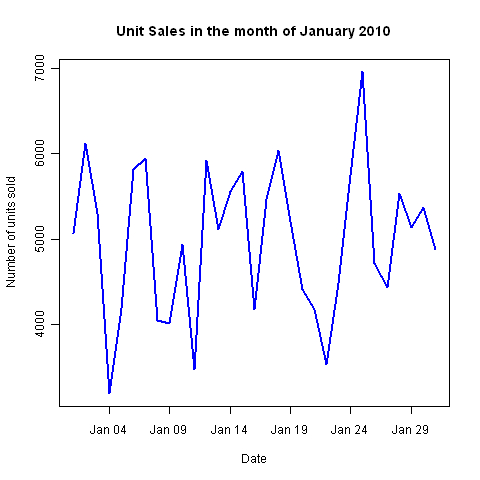### How it works...

We first read the data file using the﻿` read.csv()` function. We passed two arguments to the function: the name of the file we want to read (dailysales.csv in double quotes) and with` header=TRUE` we specified that the first row contains column headings. We read the contents of the file and saved it in an object called` sales` with the left arrow notation.

You must have noticed that the plotting code is quite similar to that for producing a scatter plot. The main difference is that this time we passed the` type` argument. The` type` argument tells the` plot()` function whether you want to plot points, lines, or other symbols. It can take nine different values.

### Note

Please see the help section on` plot()` for more details. The default value of type is`"p"` as in points.

If the type is not specified R assumes you want to plot points as it did in the scatter plot example.

The most important part of the example is the way we read the date using the` as.Date()` function. Reading dates in R is a bit tricky. R doesn't automatically recognize date formats. The﻿` as.Date()` function takes two arguments: the first is the variable which contains the date values and the second is the format the date values are stored in. In the example, the dates are in the form date/month/year or dd/mm/yyyy, which we specified as` %d/%m/%y` in the function call. If the date was in mm/dd/yyyy format, we'd use` %m/%d/%y`.

The plot and axes titles and line color are set using the same arguments as for the scatter plot.

### There's more...

If you want to plot another line on the same graph, say daily sales data of a second product, you can use the` lines()` function:

```lines(sales\$units2~as.Date(sales\$date,"%d/%m/%y"),
col`="red")
```

Line graphs and time series charts are covered in depth in Chapter 4, Creating Line Graphs and Time Series Plots.

## Creating bar charts

In this recipe, we will learn how to make bar plots, which are useful for visualizing summary data across various categories, such as sales of products or results of elections.

First we need to load the` citysales.csv` example data file. You can download this file from the code download section of the book's companion website:

```sales<-read.csv("citysales.csv",header=TRUE)
```

### How to do it...

Just like the` plot()` function we used to make scatter plots and line graphs in the earlier recipes, the` barplot()` and` dotchart()` functions are part of the base graphics library in R. This means that we don't need to install any additional packages or libraries to use these functions.

We can make bar plots using the﻿` barplot()` function as follows:

```barplot(sales\$ProductA,
names.arg= sales\$City,
col="black")
```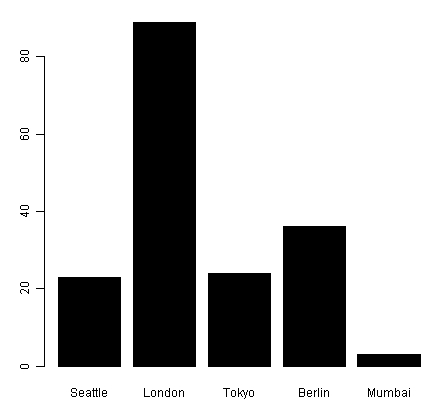The default setting of orientation for bars is vertical. To change the bars to horizontal, use the﻿` horiz` argument (by default, it is set to` FALSE):`

```barplot(sales\$ProductA,
names.arg= sales\$City,
horiz=TRUE,
col="black")
```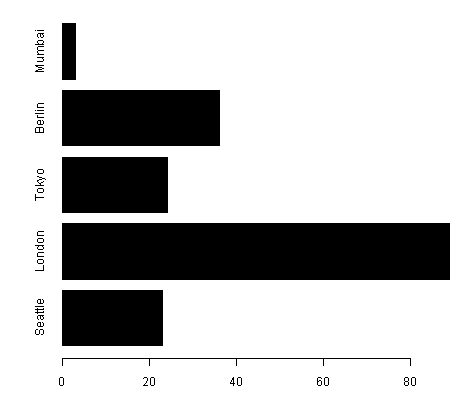### How it works...

The first argument of the` barplot()` function is either a vector or matrix of values which you want to plot as bars, such as the sales data variables in the examples we have just seen. The labels for the bars are specified by the` names.arg` argument, but we use this argument only when plotting single bars. In the example with sales figures for multiple products, we didn't specify` names.arg`. R automatically used the product names as the labels and we had to instead specify the city names as the legend.

As with the other types of plots, the` col` argument is used to specify the color of the bars. This is a common feature throughout R, that is` col` is used to set the color of the main feature in any kind of graph.

### There's more...

Bar plots are often used to compare the values of groups of values across categories. For example, we can plot the sales in different cities for more than one product using the` beside` argument﻿:

```barplot(as.matrix(sales[,2:4]), beside=TRUE,
legend=sales\$City,
col=heat.colors(5),
border="white")
```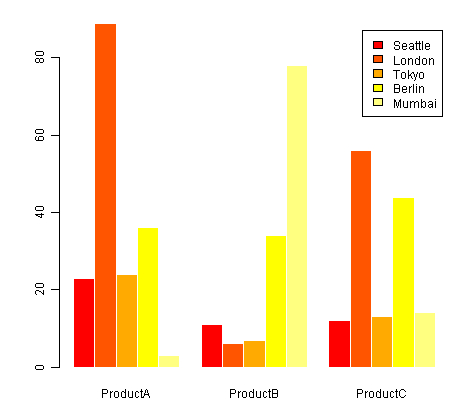You will notice that when plotting data for multiple products (columns), we used the square bracket notation in the form` sales[,2:4]`. In R the square bracket notation is used to refer to specific columns and rows of a dataset. For example,` sales[2,3]` refers to the value in the second row and the third column.

So the notation is of the form` sales[row,column]`. If you want to refer to all the rows in a certain column you can omit the row number. For example, if you want to refer to all the rows in column two, you would use` sales[,2]`. Similarly, for all the columns of row three, you would use` sales[3,]`.

So` sales[,2:4]` refers to all the data in columns two to four, which is the product sales data as shown in the following table:

City

ProductA

ProductB

ProductC

San Francisco

23

11

12

London

89

6

56

Tokyo

24

7

13

Berlin

36

34

44

Mumbai

3

78

14

The orientation of bars is set to vertical by default. It is controlled by the optional` horiz` (for horizontal) argument. If we do not use this argument in our` barplot()` function call, it is set to` FALSE`. To make the bars horizontal, we set` horiz` to` TRUE`.

The` beside` argument﻿ is used to specify whether we want the bars in a group of data to be stacked or adjacent to each other. By default,` beside` is set to` FALSE`, which produces a stacked bar graph. To make the bars adjacent, we set` beside` to` TRUE`.

To change the color of the border around the bars, we used the` border` argument. The default border color is black. But if you wish to use another color, say white, you can set it with` border="white"`.

To make the same graph with horizontal bars we would type:

```barplot(as.matrix(sales[,2:4]), beside=TRUE,
legend=sales\$City,
col=heat.colors(5),
border="white",
horiz=TRUE)
```

Bar charts will be explored in a lot more detail with some advanced recipes in Chapter 5,

## Creating histograms and density plots

In this recipe, we will learn how to make histograms and density plots, which are useful to look at the distribution of values in a dataset.

### How to do it...

The simplest way to demonstrate the use of a histogram is to show a normal distribution:

```hist(rnorm(1000))
```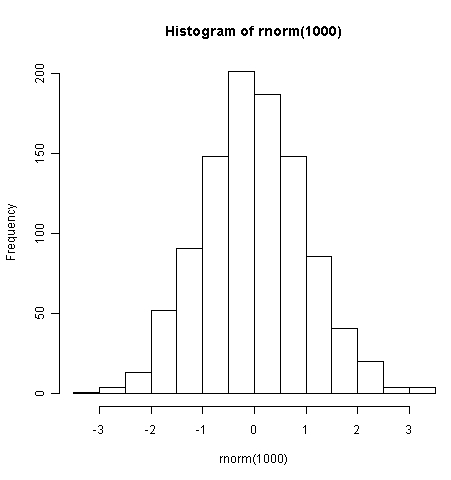Another example of a histogram is one which shows a skewed distribution:

```hist(islands)
```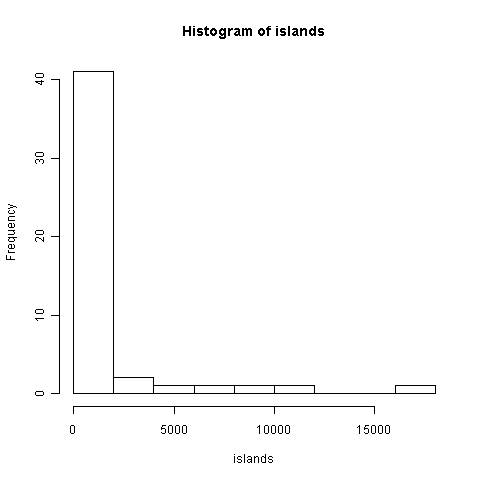### How it works...

The` hist()` function is also a function of R's base graphics library. It takes only one compulsory argument, that is the variable whose distribution of values we wish to visualize.

In the first example, we passed the﻿` rnorm()` function as the variable.` rnorm(1000)` generates a vector of 1,000 random numbers with a normal distribution. As you can see in the histogram, it's a bell-shaped curve.

In the second example, we passed the inbuilt islands dataset (which gives the areas of the world's major landmasses) as the argument to` hist()`. As you can see from that histogram, islands has a distribution skewed heavily towards the lower value range of 0 to 2,000 square miles.

### There's more...

As you may have noticed in the preceding examples, the default setting for histograms is to display the frequency or number of occurrences of values in a particular range on the Y axis. We can also display probabilities instead of frequencies by setting the` prob` (for probability) argument to` TRUE` or the` freq` (for frequency) argument to` FALSE`.

Now let's make a density plot for the same function` rnorm()`. To do so, we need to use the` density()` function and pass it as our first argument to` plot()` as follows:

```plot(density(rnorm(1000)))
```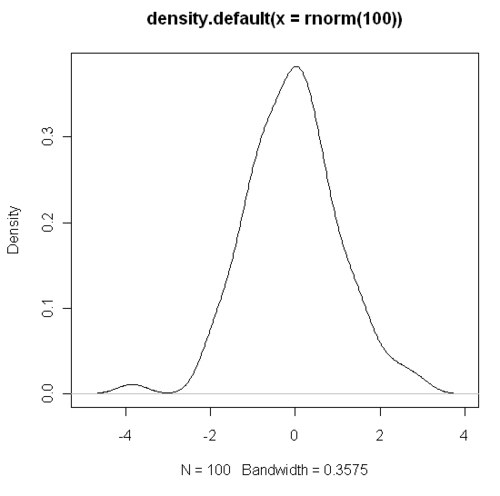We will cover more details such as setting the breaks, density, formatting of bars and other advanced recipes in Chapter 6,

## Creating box plots

In this recipe, we will learn how to make box plots, which are useful in comparing the spread of values in different measurements.

First we need to load the` metals.csv` example data file, which contains measurements of metal concentrations in London's air. You can download this file from the code download section of the book's companion website:

```metals<-read.csv("metals.csv",header=TRUE)
```

### How to do it...

We can make a box plot to summarize the metal concentration data using the﻿` boxplot()` command as follows:

```boxplot(metals,
xlab="Metals",
ylab="Atmospheric Concentration in ng per cubic metre",
main="Atmospheric Metal Concentrations in London")
```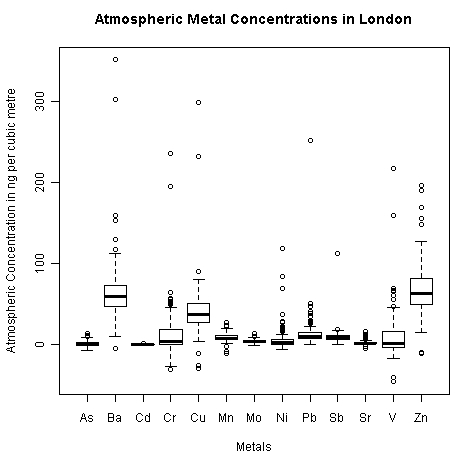### How it works...

The main argument a` boxplot()` function takes is a set of numeric values (in the form of a vector or data frame). In our first example, we used a dataset containing numerical values of air pollution data from London. The dark line inside the box for each metal represents the median of values for that metal. The bottom and top edges of the box represent the first and third quartiles respectively. Thus, the length of the box is equal to the interquartile range (IQR, difference between first and third quartiles). The maximum length of a whisker is a multiple of the IQR (default multiplier is approximately 1.5). The ends of the whiskers are at data points closest to the maximum length of the whisker.

All the points lying beyond these whiskers are considered outliers.

As with most other plot types, the common arguments such as` xlab, ylab`, and` main` can be used to set the titles for the X and Y axes and the graph itself respectively.

### There's more...

We can also make another type of box plot where we can group the observations by categories. For example, if we want to study the spread of copper concentrations by the source of the measurements, we can use a formula to include the source. First we need to read the` copper_site.csv` example data file, as follows:

```copper<-read.csv("copper_site.csv",header=TRUE)
```

Then we can add the following code:

```boxplot(copper\$Cu~copper\$Source,
xlab="Measurement Site",
ylab="Atmospheric Concentration of Copper in ng per cubic metre",
main="Atmospheric Copper Concentrations in London")
```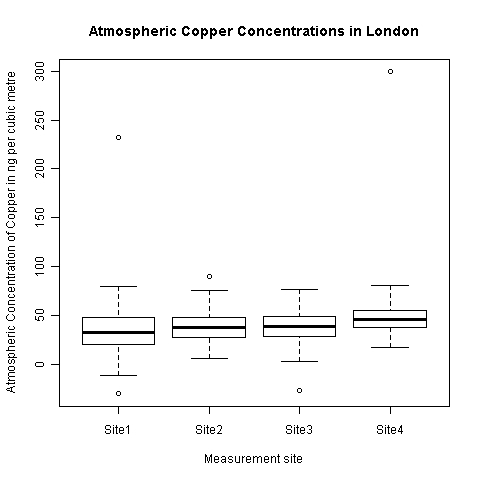In this example, the` boxplot()` function takes a formula as an argument. This formula in the form` value~group` (Cu~source) specifies a column of values and the group of categories it should be summarized over.

More detailed box plot recipes will be presented in Chapter 7, Creating Box and Whisker Plots.

## Adjusting X and Y axes limits

In this recipe, we will learn how to adjust the X and Y limits of plots, which is useful in adjusting a graph to suit one's presentation needs and adding additional data to the same plot.

### How to do it...

We will modify our first scatter plot example to demonstrate how to adjust axes limits:

```plot(cars\$dist~cars\$speed,
xlim=c(0,30),
ylim=c(0,150))
```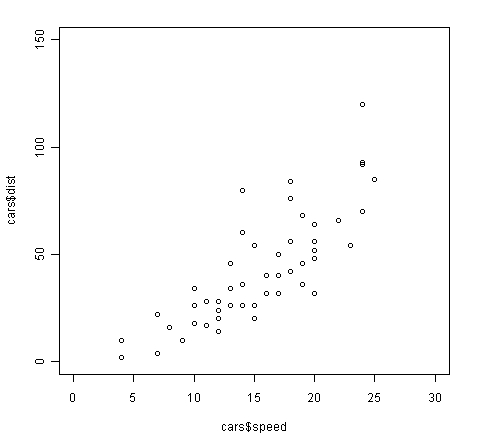### How it works...

In our original scatter plot in the first recipe of this chapter, the x axis limits were set to just below 5 and up to 25 and the y axis limits were set from 0 to 120. In this example, we set the x axis limit to 0 to 30 and y axis limits to 0 to 150 using the` xlim` and` ylim` arguments respectively.

Both` xlim` and` ylim` take a vector of length 2 as valid values in the form` c(minimum,maximum)that` is,` xlim=c(0,30)` means set the x axis minimum limit to 0 and maximum limit to 30.

### There's more...

You may have noticed that even after setting the x and y limit values, there is some gap left at either edges. The two axes zeroes don't coincide. This is because R automatically adds some additional space at both the edges of the axes, so that if there are any data points at the extremes, they are not cut off by the axes. If you wish to set the axes limits to exact values, in addition to specifying` xlim` and` ylim`, you must also set the` xaxs` and` yaxs` arguments to`"i":`

```plot(cars\$dist~cars\$speed,
xlim=c(0,30),
ylim=c(0,150),
xaxs="i",
yaxs="i")
```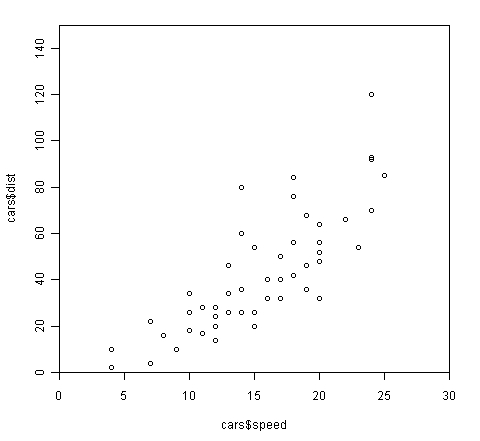Sometimes, we may wish to reverse a data axis, say to plot the data in descending order along one axis. All we have to do is swap the minimum and maximum values in the vector argument supplied as` xlim` or` ylim`. So, if we want the X axis speed values in the previous graph in descending order we need to set` xlim` to` c(30,0):`

```plot(cars\$dist~cars\$speed,
xlim=c(30,0),
ylim=c(0,150),
xaxs="i",
yaxs="i")
```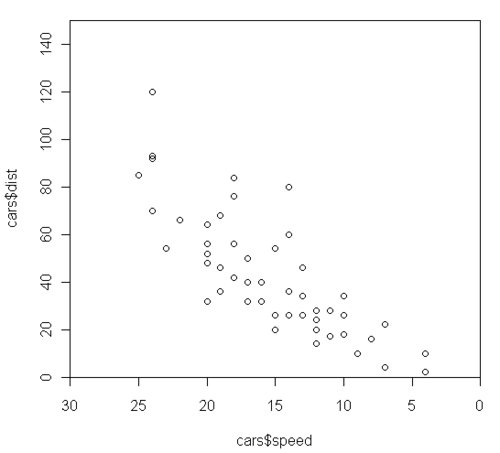There will be a few more recipes on adjusting the axes tick marks and labels in Chapter 2,

## Creating heat maps

Heat maps are colorful images, which are very useful for summarizing a large amount of data by highlighting hotspots or key trends in the data.

### How to do it...

There are a few different ways to make heat maps in R. The simplest is to use the﻿` heatmap()` function in the base library:

```heatmap(as.matrix(mtcars),
Rowv=NA,
Colv=NA,
col = heat.colors(256),
scale="column",
margins=c(2,8),
main = "Car characteristics by Model")
```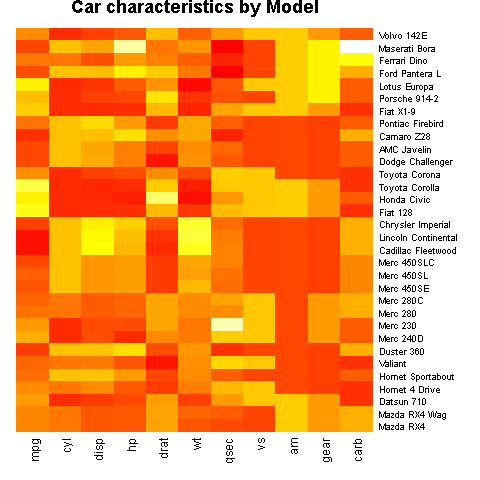### How it works...

The example code has a lot of arguments, so it may look difficult at first sight. But if we consider each argument in turn, we can understand how it works. The first argument to the﻿` heatmap()` function is the dataset. We are using the inbuilt dataset` mtcars`, which holds data such as fuel efficiency (mpg), number of cylinders (cyl), weight (wt), and so on for different models of cars. The data needs to be in a matrix format, so we use the` as.matrix()` function.` Rowv` and` Colv` specify if and how dendrograms should be displayed to the left and top of the heat map.

### Note

See` help(dendrogram)` and http://en.wikipedia.org/wiki/Dendrogram for details on dendrograms.

In our example, we suppress them by setting the two arguments to` NA`, which is a logical indicator of a missing value in R. The` scale` argument tells R in what direction the color gradient should apply. We have set it to column, which means the scale for the gradient will be calculated on a per-column basis.

### There's more...

Heat maps are very useful for looking at correlations between variables in a large dataset. For example, in bioinformatics, heat maps are often used to study the correlations between groups of genes.

Let's look at an example with the` genes.csv` example data file. Let's first load the file:

```genes<-read.csv("genes.csv",header=T)
```

Let's use the` image()` function to create a correlation heat map:

```rownames(genes)<-colnames(genes)
image(x=1:ncol(genes),
y=1:nrow(genes),
z=t(as.matrix(genes)),
axes=FALSE,
xlab="",
ylab="" ,
main="Gene Correlation Matrix")
axis(1,at=1:ncol(genes),labels=colnames(genes),col="white",
las=2,cex.axis=0.8)
axis(2,at=1:nrow(genes),labels=rownames(genes),col="white",
las=1,cex.axis=0.8)
```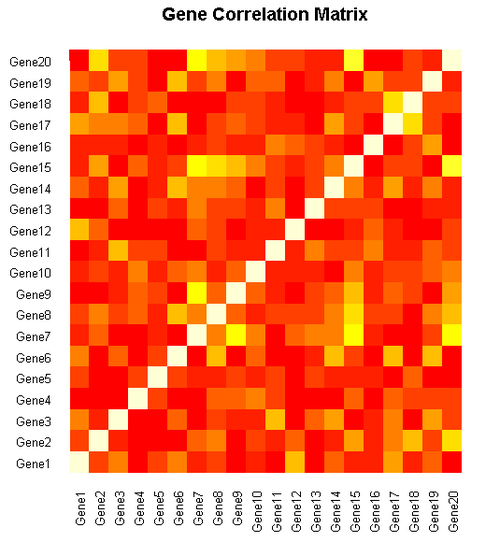We have used a few new commands and arguments in this example, especially for formatting the axes. We will discuss these in detail starting in Chapter 2, Beyond the Basics and with more examples in later chapters.

Heat maps will be explained in a lot more detail with more examples in Chapter 8,

## Creating pairs plots

A pairs plot is a matrix of scatter plots which is a very handy visualization for quickly scanning the correlations between many variables in a dataset.

### How to do it...

We will use the inbuilt` iris` dataset, which gives the measurements in centimeters of the variables sepal length, sepal width, petal length and petal width, respectively, for 50 flowers from each of three species of iris:

```pairs(iris[,1:4])
```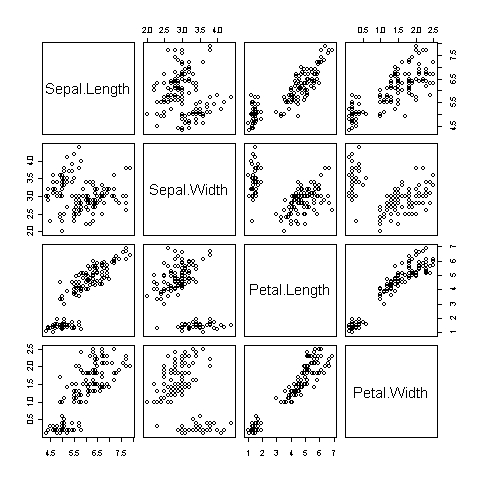### How it works...

As you can see in the figure, the﻿` pairs()` command makes a matrix of scatter plots, where all the variables in the specified dataset are plotted against each other. The variable names, displayed in the diagonal running across from the top left to the bottom right, are the key to reading the graph. For example, the scatter plot in the first row and second column shows the relationship between Sepal Length on the Y axis and Sepal Width on the X axis.

### There's more...

Here's a fun fact: we can produce the previous graph using the` plot()` function instead of` pairs()` in exactly the same manner:

```plot(iris[,1:4],
main="Relationships between characteristics of iris flowers",
pch=19,
col="blue",
cex=0.9)
```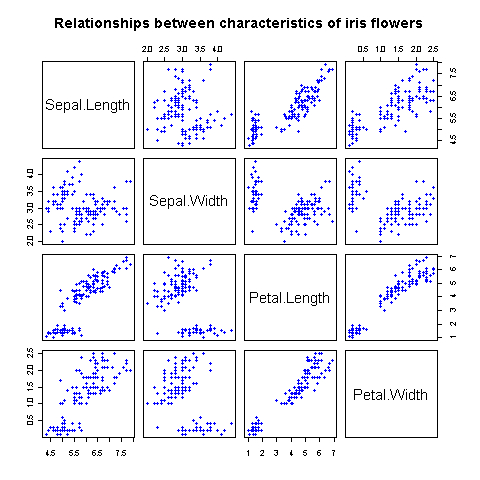So if you pass a data frame with more than two variables to the` plot()` function, it creates a scatter plot matrix by default. We've also added a plot title and modified the plotting symbol style, color and size using the` pch, col` and` cex` arguments respectively. We'll delve into the details of these settings in Chapter 2,

We'll cover some more interesting recipes in Chapter 3, Creating Scatter Plots, building upon the things we learn in Chapter 2.

## Creating multiple plot matrix layouts

In this recipe, we will learn how to present more than one graph in a single image. Pairs plots are one example as we saw in the last recipe, but here we will learn how to include different types of graphs in each cell of a graph matrix.

### How to do it...

Let's say we want to make a 2x3 matrix of graphs, made of two rows and three columns of graphs. We use the` par()` command as follows:

```par(mfrow=c(2,3))
plot(rnorm(100),col="blue",main="Plot No.1")
plot(rnorm(100),col="blue",main="Plot No.2")
plot(rnorm(100),col="green",main="Plot No.3")
plot(rnorm(100),col="black",main="Plot No.4")
plot(rnorm(100),col="green",main="Plot No.5")
plot(rnorm(100),col="orange",main="Plot No.6")
```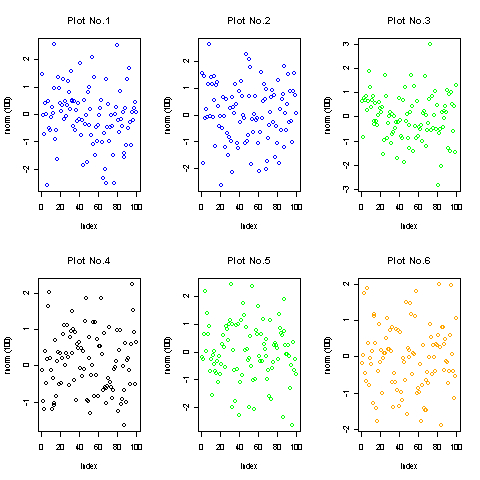### How it works...

The` par()` command is by far the most important function for customizing graphs in R. It is used to set and query many graphical arguments (hence par), which control the layout and appearance of graphs.

Please note that we need to issue the` par()` command before the actual graph commands. When you first run the` par()` command, only a blank graphics window appears. The` par()` command sets the argument for any subsequent graphs made. The` mfrow` argument is used to specify how many rows and columns of graphs we wish to plot. The` mfrow` argument takes values in the form of a vector of length two:` c(nrow,ncol)`. The first number specifies the number of rows and the second specifies the number of columns. In our previous example, we wanted a matrix of two rows and three columns, so we set` mfrow` to` c(2,3)`.

Note that there is another argument` mfcol`, similar to` mfrow`, which can also be used to create multiple plot layouts.` mfcol` also takes a two value vector specifying the number of rows and columns in the matrix. The difference is that` mfcol` draws subsequent figures by columns, rather than by rows as` mfrow` does. So, if we used` mfcol` instead of` mfrow` in the earlier example, we would get the following plot: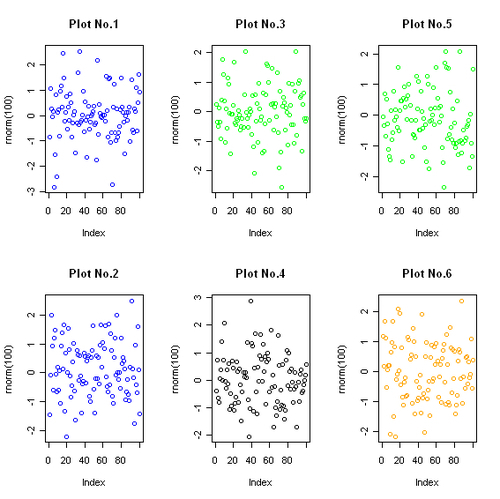### There's more...

Let's look at a practical example where a multiple plot layout would be useful. Let's read the` dailymarket.csv` example file that contains data on the daily revenue, profits, and number of customer visits for a shop:

```market<-read.csv("dailymarket.csv",header=TRUE)
```

Now, let's plot all the three variables over time in a plot matrix with the graphs stacked over one another:

```par(mfrow=c(3,1))
plot(market\$revenue~as.Date(market\$date,"%d/%m/%y"),
type="l", #Specify type of plot as l for line
main="Revenue",
xlab="Date",
ylab="US Dollars",
col="blue")
plot(market\$profits~as.Date(market\$date,"%d/%m/%y"),
type="l", #Specify type of plot as l for line
main="Profits",
xlab="Date",
ylab="US Dollars",
col="red")
plot(market\$customers~as.Date(market\$date,"%d/%m/%y"),
type="l", #Specify type of plot as l for line
main="Customer visits",
xlab="Date",
ylab="Number of people",
col="black")
```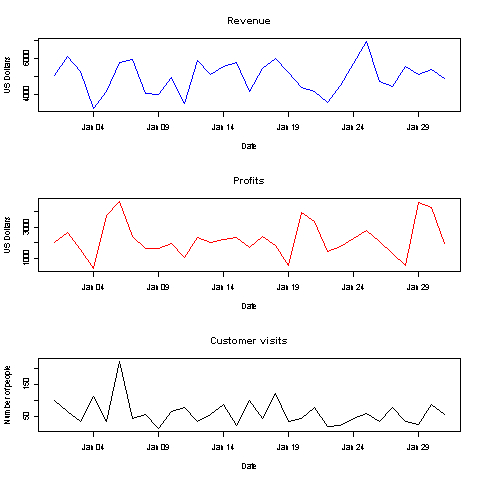The preceding graph is a good way to visualize variables with different value ranges over the same time period. It helps in identifying where the trends match each other and where they differ.

We will explore more examples and uses of multiple plot layouts in later chapters.

In this recipe, we will learn how to add and format legends to graphs.

First we need to load the` cityrain.csv` example data file, which contains monthly rainfall data for four major cities across the world. You can download this file from the code download section of the book's companion website:

```rain<-read.csv("cityrain.csv",header=TRUE)
```

### How to do it...

In the bar plots recipe, we already saw that we can add a legend by passing the` legend` argument to the` barplot()` function. Now we see how we can use the` legend()` function to add and customize a legend for any type of graph.

Let's first draw a graph with multiple lines representing the rainfall in cities:

```plot(rain\$Tokyo,type="l",col="red",
ylim=c(0,300),
main="Monthly Rainfall in major cities",
xlab="Month of Year",
ylab="Rainfall (mm)",
lwd=2)
lines(rain\$NewYork,type="l",col="blue",lwd=2)
lines(rain\$London,type="l",col="green",lwd=2)
lines(rain\$Berlin,type="l",col="orange",lwd=2)
```

Now let's add the legend to mark which line represents which city:

```legend("topright",
legend=c("Tokyo","NewYork","London","Berlin"),
col=c("red","blue","green","orange"),
lty=1,lwd=2)
```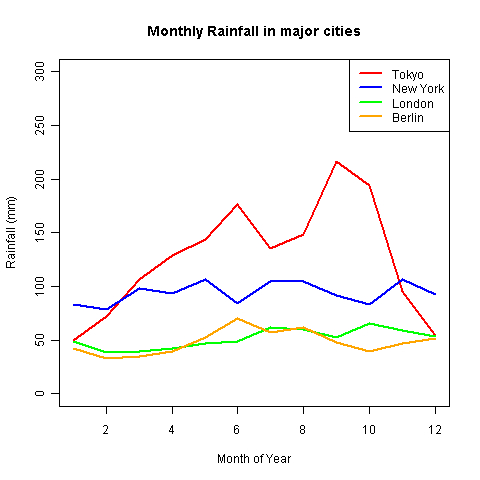### How it works...

In the example code, we first created a graph with multiple lines using the` plot()` and` lines()` commands to represent the monthly rainfall in Tokyo, New York, London, and Berlin in four different colors. However, without a legend one would have no way of telling which line represents which city. So we added a legend using the` legend()` function﻿.

The first argument to the` legend()` function is the position of the legend, which we set to` topright`. Other possible values are`"topleft", "top", "left", "center", "right", "bottomleft", "bottom"`, and`"bottomright"`. Then we specify the legend labels by setting the` legend` argument to a vector of length 4 containing the names of the four cities. The` col` argument specifies the colors of the legend, which should match the colors of the lines in exactly the same order. Finally, the line type and width inside the legend are specified by` lty` and` lwd` respectively.

### There's more...

The placement and look of the legend can be modified in several ways. As a simple example, let's spread the legend across the top of the graph instead of the top right corner. So first, let's redraw the same base plot:

```plot(rain\$Tokyo,type="l",col="red",
ylim=c(0,250),
main="Monthly Rainfall in major cities",
xlab="Month of Year",
ylab="Rainfall (mm)",
lwd=2)
lines(rain\$NewYork,type="l",col="blue",lwd=2)
lines(rain\$London,type="l",col="green",lwd=2)
lines(rain\$Berlin,type="l",col="orange",lwd=2)
```

Now, let's add a modified legend:

```legend("top",
legend=c("Tokyo","NewYork","London","Berlin"),
ncol=4,
cex=0.8,
bty="n",
col=c("red","blue","green","orange"),
lty=1,lwd=2)
```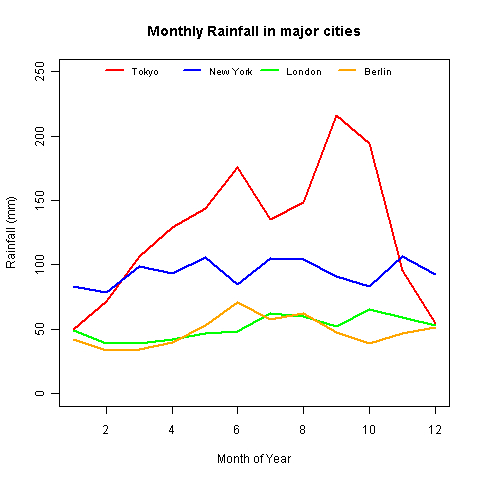We changed the legend location from` topright` to` top` and added a few other arguments to adjust the look. The` ncol` argument is used to specify the number of columns over which the legend is displayed. The default value is` 1` as we saw in the first example. In our second example, we set` ncol` to` 4` so that all the city names are displayed in one single row. The argument` bty` specifies the type of box drawn around the legend. We removed it from the graph by setting it to`"n"`. We also modified the size of the legend labels by setting` cex` to` 0.8`.

There are plenty of examples of how you can add and customize legends in different scenarios in later chapters.

## Creating graphs with maps

In this recipe, we will learn how to plot data on maps.

In order to plot maps in R, we need to install the maps library. Here's how to do it:

```install.packages("maps")
```

When you run this command, you will most likely be prompted by R to choose from a list of locations from where you can download the library. For example, if you are based in the UK, you can choose either the UK (Bristol) or UK (London) options.

Once the library is installed, we must load it using the` library()` command:

```library(maps)
```

### Note

Note that we need to install any package using` install.packages()` only once but need to load it using` library()` or` require()` every time we restart a new session in R.

### How to do it...

We can make a simple world map with just one command:

```map()
```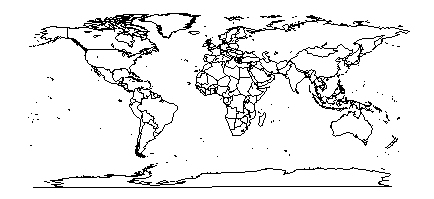```map('world', fill = TRUE,col=heat.colors(10))
```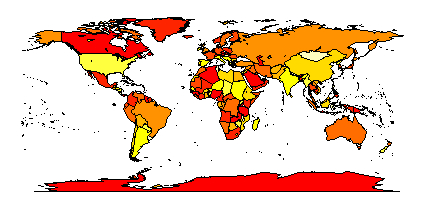### How it works...

The` maps` library provides a way to project world data on to a low resolution map. It is also possible to make detailed maps of the United States. For example, we can make a map showing the state boundaries as follows:

```map("state", interior = FALSE)
map("state", boundary = FALSE, col="red", add = TRUE)
```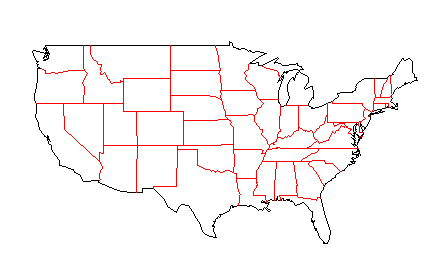The` add` argument is set to` TRUE` in the second call to` map()` to add details to the same map created using the first call. It only works if a map has already been drawn on the current graphic device.

### There's more...

The previous examples are just a basic introduction to the idea of geographical visualization in R. In order to plot any useful data, we need to use a better maps library. GADM (http://gadm.org) is a free spatial database of the location of the world's administrative areas (or administrative boundaries). The site provides map information as native R objects that can be plotted directly with the use of the` sp` library.

Let's take a look at a quick example. First we need to install and load the` sp` library, just like we did with the` maps` library:

```install.packages("sp")
library(sp)
```

GADM provides data for all the countries across the world. Let's load the data for Great Britain. We can do so by directly reading the data from the GADM website:

```load(url("http://gadm.org/data/rda/GBR_adm1.RData"))
```

This command loads the boundary data for the group of administrative regions forming Great Britain. It is stored in memory as a data object named` gadm`. Now let's plot a map with the loaded data:

```spplot(gadm,"Shape_Area")
```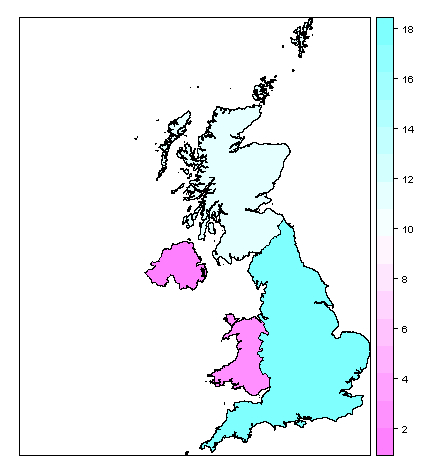The graph shows the different parts of Great Britain, color coded by their surface areas. We could just as easily display any other data such as population or crime rates.

We will cover more detailed and practical recipes with maps in Chapter 9,

## Saving and exporting graphs

In this recipe, we will learn how to save and export our graphs to various useful formats.

### How to do it...

To save a graph as an image file format such as PNG, we can use the` png()` command:

```png("scatterplot.png")
plot(rnorm(1000))
dev.off()
```

The preceding command will save the graph as` scatterplot.png` in the current working directory. Similarly, if we wish to save the graph as JPEG, BMP or TIFF we can use the` jpeg(), bmp()`, or` tiff()` commands respectively.

If you are working under Windows, you can also save a graph using the graphical user interface. First make your graph, make sure the graph window is the active window by clicking anywhere inside it and then click on File | Save as | Png or the format of your choice as shown in the following screenshot: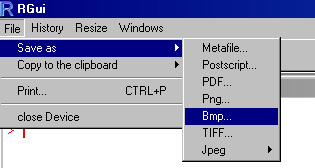When prompted to choose a name for your saved file, type a suitable name and click Save. As you can see, you can choose from 7 different formats.

### How it works...

If you wish to use code to save and export your graphs, it is important to understand how the code works. The first step in saving a graph is to open a graphics device suitable for the format of your choice before you make the graph. For example, when you call the` png()` function, you are telling R to start the PNG graphics device, such that the output of any subsequent graph commands you run will be directed to that device. By default, the display device on the screen is active. So any graph commands result in showing the graph on your screen. But you will notice that when you choose a different graphics device such as` png()`, the graphs don't show up on your screen. Finally, you must close the graphics device with the` dev.off()` command to instruct R to save the graph you plotted in the specified format and write it to disk with the specified filename. If you do not run` dev.off()`, the file will not be saved.

### There's more...

You can specify a number of arguments to adjust the graph as per your needs. The simplest one that we've already used is the filename. You can also adjust the height and width settings of the graph:

```png("scatterplot.png",
height=600,
width=600)
```

The default units for height and width are pixels but you can also specify the units in inches, cm or mm:

```png("scatterplot.png",
height=4,
width=4,
units="in")
```

The resolution of the saved image can be specified in dots per inch (dpi) using the` res` argument:

```png("scatterplot.png",
res=600)
```

If you want your graphs saved in a vector format, you can also save them as a PDF file using the` pdf()` function:

```pdf("scatterplot.pdf")
```

Besides maintaining a high resolution of your graphs independent of size, PDFs are also useful because you can save multiple graphs in the same PDF file.